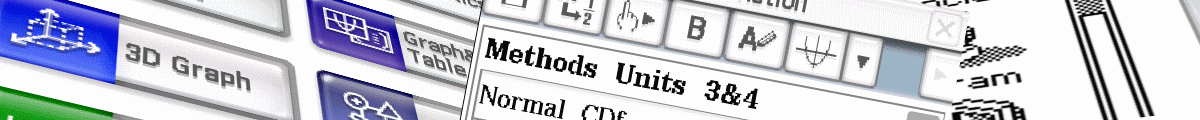Want help to update your ClassPad OS? Methods & Spec students - beware of bugs.

eActivities are a great way to see how you can use your ClassPad to go beyond the basics. eActivities can be downloaded and sent to your handheld using the USB cable and free software that came with your ClassPad. Learn to make an eActivity yourself using our helpsheets for either ClassPad II or ClassPad. Also visit Classpad Help Series and watch videos on eActivities.

Cascade type eActivities. Nearly all the eActivities below are re-usable templates for carrying out a serie s of steps to solve a problem. I think of them as 'cascade' type eActivities, as you simply modify some of the initial variables, tap EXE and the whole problem is re-calculated step by step from top to bottom.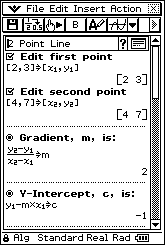2PointLine At the top of this eActivity file, change the coordinates of two points, tap EXE and watch as just about everything you want - equation of line through points, distance between them, midpoint, perpendicular gradient, etc is calculated. As a bonus, all the formulas used are clearly visible and just like those found in most text books - so you can see how everything is calculated!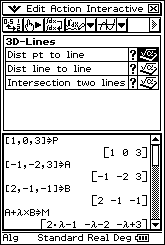3D-Lines has a couple of main strips used for 3 dimensional vector line problems - (a) finding the distance from a point to a line and (b) finding the point of interscetion (if it exists) of two lines.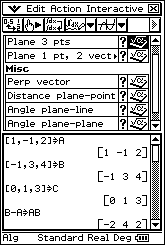3D-Planes has strips used for 3 dimensional vector plane problems - (a) equation of plane from three points (b) equation of plane from one point and two vectors in the plane (c) finding point of intersection of line and plane (d) finding a vector perpendicular to a given vector (e) distance from a point to a plane (f) angle between a line and a plane.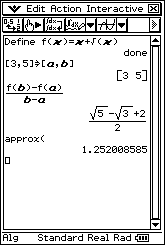AvgRateChange A Main strip saved in an eActivity for calculating the average rate of change of a function between x=a and x=b. Simply modify f(x) and the values of a and b and tap EXE. If the Main strip is set to Standard mode, then approx() automatically gives a decimal approximation to the exact value.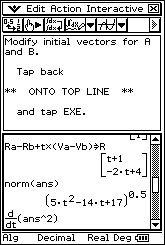ClosestAppCalc A Main strip saved as an eActivity to determine the time and position of the closest approach of two moving points A and B using a mixture of vector and calculus methods. Simply modify the initial position and velocity vectors for A and B and tap EXE. Works great for both 2D and 3D vectors - just add or remove the k coefficent as required. All the expresions are re-calculated and you have plenty of working to copy onto your test paper!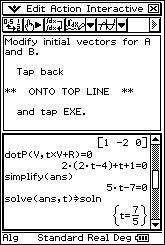ClosestAppDot Similar to the previous eActivity, this is a Main strip saved as an eActivity to determine the time and position of the closest approach of two moving points A and B, only in this case using purely vector methods. Simply modify the initial position and velocity vectors for A and B and tap EXE. Works great for both 2D and 3D vectors - just add or remove the k coefficent as required. All the expresions are re-calculated and you have plenty of working to copy onto your test paper!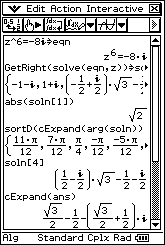ComplexSolver is a single strip to help tidy up the solutions to complex equations. Often the solutions are way too wide for the screen and not returned in a very tidy form (try the equation shown in the screen dump and look at the fourth and fifth solns - uuggh!). This eActivity presents the modulus of the solution on the third line and displays the arguments in descending order on the fourth line. To view the entire nth solution, modify soln to soln[n].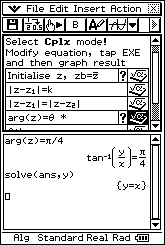ComplexRegions has a collection of strips to help deal with graphing complex numbers on an Argand diagram.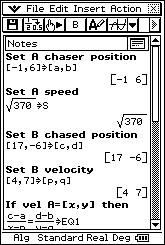CourseToIntercept This eActivity uses a mixture of text and calculation lines to determine the time and velocity vector required by A to catch B, when all we know is the maximum speed of A. Simply modify the initial conditions for A and B and tap EXE. All the expresions are re-calculated and you have plenty of working to copy onto your test paper!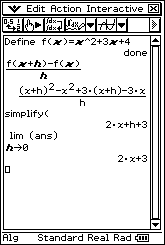DiffFirstPrinc A Main strip saved in an eActivity for finding the derivative of a function from first principles. Simply modify f(x), tap EXE and copy down some working for full marks!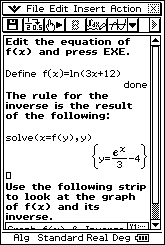InverseFunctions is a simple, but very useful eActivity, to help with finding and graphing inverse functions.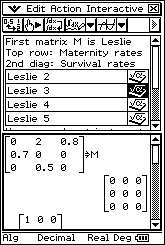LeslieMatrices contains four templates ready to enter coefficients for Leslie matrix problems involving 2, 3, 4 or 5 generations.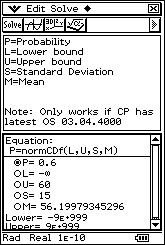NormalCalcs Use the normCDF function in a NumSolve strip to solve for any of the variables, including the mean and standard deviation.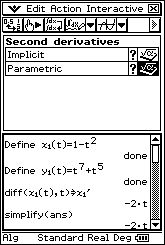SecondDiffs is useful for finding second (and first) derivatives of functions defined implicitly or parametrically. Simply modify initial function, tap EXE and copy down some working for full marks!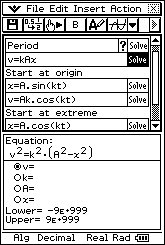SHM has a collection of solve strips to help deal with simple harmonic motion problems.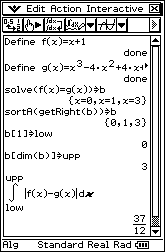TrappedArea Modify the two functions f(x) and g(x) at the top of this Main strip and tap EXE. The strip finds all solutions to f(x)=g(x), sorts to find and set the limits of integration and the trapped area is calculated. Did that take five seconds? Too easy!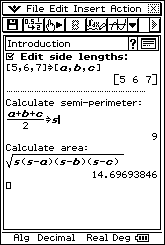TriArea3Sides Use this eActivity to find the area of a triangle when all 3 side lengths are known. Edit the 3 side lengths and instantly the semi-perimeter and area are calculated and displayed. Both formulas used are clearly visible so you can see how everything is calculated!